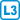## AG inequality

Prove this inequality between the geometric and arithmetic means of a set of numbers.

For non-negative $$x_1,…,x_n$$: $$\displaystyle \sqrt[n]{x_1x_2\cdots x_n} \le \frac{x_1+x_2+…+x_n}n$$

• #### Variant 1

For $$n=2$$.

• #### Variant 2

When $$n$$ is a power of two.

• #### Variant 3

For any $$n\in \mathbb N$$.